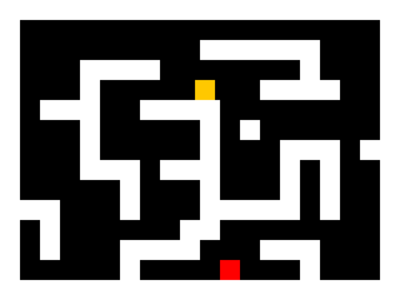# Rect Collision Response

A simple demo on how to do side-based rect collision response.pymike
(pymike)
This is a simple demo on how to do side-based rectangle collision response. It's extremely simple, and not very hard to understand.

### Concept

I'm putting this here since it's not in the download. Basically how it works is when you move a rect, you first move along the X axis, test for a collision, move out, then move along the Y axis, test for a collision, and move out. This prevents the infamous "corner-catching" bug, and lets you move smoothly along walls.```#! /usr/bin/env python

import os
import random
import pygame

# Class for the orange dude
class Player(object):

def __init__(self):
self.rect = pygame.Rect(32, 32, 16, 16)

def move(self, dx, dy):

# Move each axis separately. Note that this checks for collisions both times.
if dx != 0:
self.move_single_axis(dx, 0)
if dy != 0:
self.move_single_axis(0, dy)

def move_single_axis(self, dx, dy):

# Move the rect
self.rect.x += dx
self.rect.y += dy

# If you collide with a wall, move out based on velocity
for wall in walls:
if self.rect.colliderect(wall.rect):
if dx > 0: # Moving right; Hit the left side of the wall
self.rect.right = wall.rect.left
if dx < 0: # Moving left; Hit the right side of the wall
self.rect.left = wall.rect.right
if dy > 0: # Moving down; Hit the top side of the wall
self.rect.bottom = wall.rect.top
if dy < 0: # Moving up; Hit the bottom side of the wall
self.rect.top = wall.rect.bottom

# Nice class to hold a wall rect
class Wall(object):

def __init__(self, pos):
walls.append(self)
self.rect = pygame.Rect(pos, pos, 16, 16)

# Initialise pygame
os.environ["SDL_VIDEO_CENTERED"] = "1"
pygame.init()

# Set up the display
pygame.display.set_caption("Get to the red square!")
screen = pygame.display.set_mode((320, 240))

clock = pygame.time.Clock()
walls = [] # List to hold the walls
player = Player() # Create the player

# Holds the level layout in a list of strings.
level = [
"WWWWWWWWWWWWWWWWWWWW",
"W                  W",
"W         WWWWWW   W",
"W   WWWW       W   W",
"W   W        WWWW  W",
"W WWW  WWWW        W",
"W   W     W W      W",
"W   W     W   WWW WW",
"W   WWW WWW   W W  W",
"W     W   W   W W  W",
"WWW   W   WWWWW W  W",
"W W      WW        W",
"W W   WWWW   WWW   W",
"W     W    E   W   W",
"WWWWWWWWWWWWWWWWWWWW",
]

# Parse the level string above. W = wall, E = exit
x = y = 0
for row in level:
for col in row:
if col == "W":
Wall((x, y))
if col == "E":
end_rect = pygame.Rect(x, y, 16, 16)
x += 16
y += 16
x = 0

running = True
while running:

clock.tick(60)

for e in pygame.event.get():
if e.type == pygame.QUIT:
running = False
if e.type == pygame.KEYDOWN and e.key == pygame.K_ESCAPE:
running = False

# Move the player if an arrow key is pressed
key = pygame.key.get_pressed()
if key[pygame.K_LEFT]:
player.move(-2, 0)
if key[pygame.K_RIGHT]:
player.move(2, 0)
if key[pygame.K_UP]:
player.move(0, -2)
if key[pygame.K_DOWN]:
player.move(0, 2)

# Just added this to make it slightly fun ;)
if player.rect.colliderect(end_rect):
raise SystemExit, "You win!"

# Draw the scene
screen.fill((0, 0, 0))
for wall in walls:
pygame.draw.rect(screen, (255, 255, 255), wall.rect)
pygame.draw.rect(screen, (255, 0, 0), end_rect)
pygame.draw.rect(screen, (255, 200, 0), player.rect)
pygame.display.flip()
```

### Changes